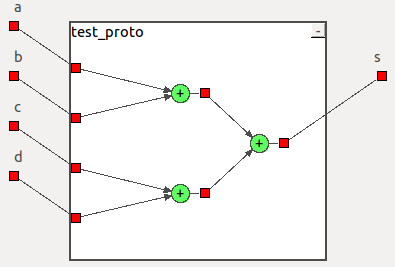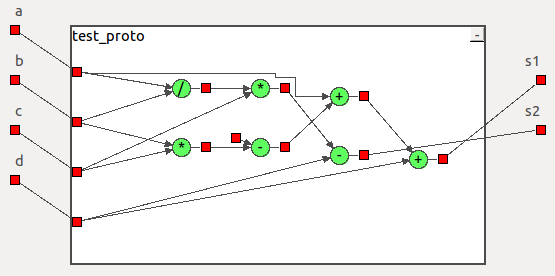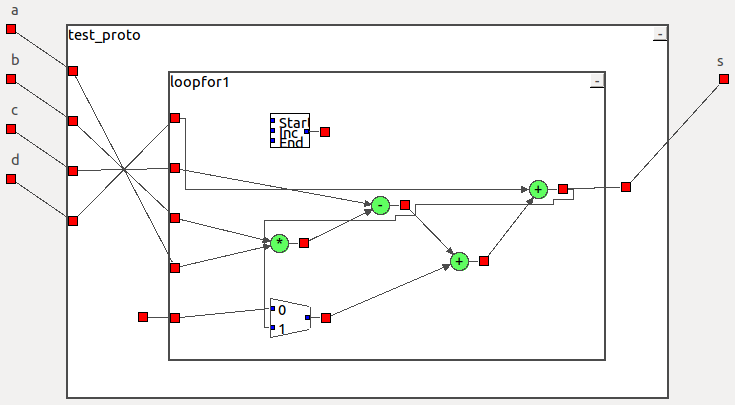# Examples

First of all, here is a small video demonstrating the tool:

Here are some examples illustrating the accepted syntax. Each example is followed by an illustration of the corresponding hardware structure (generated by the tool).

```function s = test_proto(a,b,c,d)
s=a+b+c+d;
endfunction``````function s = test_proto(a,b,c,d)
s=(a+b)+(c+d);
endfunction``````function [s1,s2]=test_proto(a,b,c,d)
s1=a+(b*c-10)+d;
s2=a/b*c-d;
endfunction``````function s=test_proto(a,b,c,d)
s = 10;
for i=1:1:10
s = s+a*b-c+d;
end
endfunction``````function  s = test_proto(a,b)
if (a<b)
s=a+b;
else
s=a-b;
endif
endfunction``````function  s = test_proto(a,b,c,d)
tmp = c+d*a;
if(c>d)
if(c>17)
s = a+tmp;
else
s = b+2*tmp;
end
else
if(c>6)
s = a+tmp;
else
s = b+a*tmp;
end
end
endfunction```